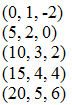# Lines in Space

## Objective

SWBAT to write the parametric equations and symmetric equation for a line in three dimensions.

#### Big Idea

Parametric equations are back! This time they will help us write the equation of a 3D line.

## Launch and Explore

15 minutes

I like today's lesson because it brings back a topic that we learned in a past unit, but in an unexpected context. Students will use parametric equations to allow us to write an equation of a line in three dimensions.

Students have so much experience with equations of lines, but looking at them in 3D may throw them off. I try to build a little conceptual understanding of lines in 3D and the nature of their slopes in order to build a bridge to finding their equations.

I get started by showing students slide 2 of this PowerPoint and have them name three other points that are on a line given by two points. The purpose of this is to get them thinking about the slope and how the x, y, and z coordinates must increase or decrease in the same ratio. Students are also asked to describe the slope. Obviously they cannot write it as a single fraction, but they can at least say how the x, y, and z are changing. I usually give students 10 minutes to think about these questions and how they could generalize an equation to represent any point on the line.

## Share

20 minutes

I don't expect all of my students to be able to figure out the need to use parametric equations to write the equation of a line, but I can get them there by framing the discussion in a specific way. For slide #2 of the PowerPoint, students were given two points on the line and were asked to find three more. I usually start by writing down the first two points and then extending the pattern to add more. The list will look like this:Students will see that the x, y, and z coordinates all have their own pattern and it is related to the slope of this 3D line. If I noticed a student using parametric equations, I will have them share their thinking. If the class needs a little nudge, I will ask them to just make an equation to represent the pattern for the x-coordinates. Usually many students can describe the x pattern with an equation and I will ask them to show their work. Then, I will have them finish up the equations for y and z.

It is interesting that students will not all get a unique equation for the line - we can write many different equations to represent the same line. In this image you can see three possibilities - the first two are very obvious but the third one is not. I wrote the third set of parametric equations to see if students could make sense of why it would produce the same line as the other two sets. I talk more about this in the video below.

Next I move on to slide #3 and have students find the parametric equations for the line passing through the two new points. I will choose a few students to share their different sets of equations and we will talk about what is the same about all of the parametric equations and the reason for it. Next, I will demonstrate how we can solve each parametric equation for t and set them all equal to each other to produce the symmetric equation for the line.

## Extend and Summarize

15 minutes

The last slide of this PowerPoint gives one more problem but it is slightly different - students will have to find the equation of a line given a point and a parallel vector. Students are also challenged the write the equation as parametric equations and as a symmetric equation. I will end the lesson by having a student explain their work.

Finally, I will assign a homework assignment from the textbook to summarize what we learned.